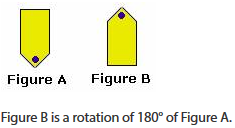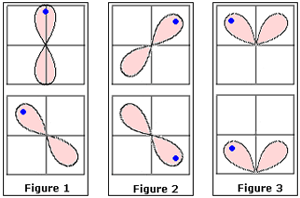## Definition Of Rotation

A Rotation is a transformation that turns a figure about a fixed point.

Rotation is also called as turn
The fixed point around which a figure is rotated is called as centre of rotation

### Example of Rotation### Solved Example on Rotation

#### Ques: Identify the figures that represent a rotation.##### Choices:

A. Figure 1 and Figure 2
B. Figure 1, Figure 2, and Figure 3
C. Figure 3 and Figure 2
D. Figure 1 and Figure 3